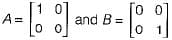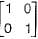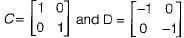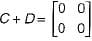Consider the following statements:
S1: The sum of two singular n x n matrices may be non-singular
S2: The sum of two n x n non-singular matrices may be singular
Which of the following statements is correct?
• a)
S1 and S2 are both true
• b)
S1 is true, S2 is false
• c)
S1 is flase, S2 is true
• d)
S1 and S2 are both false

### Related TestKamla Devi Jul 21, 2020S1 is true
Consider two singular matricesSum of A and B is given as
However (A + B) is a non-singuiar matrixSo, S1 is true.
Now, consider two non-singuiar matricesHowever (C + D) is a singular matrix. So, S2 is also true.
Therefore, both S1 and S2 are true.

S1 is trueConsider two singular matricesSum of A and B is given asHowever (A + B) is a non-singuiar matrixSo, S1 is true.Now, consider two non-singuiar matricesHowever (C + D) is a singular matrix. So, S2 is also true.Therefore, both S1 and S2 are true.